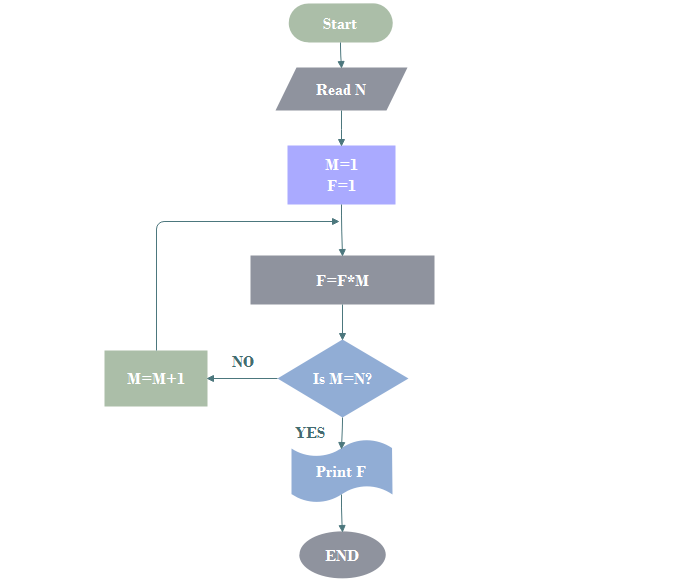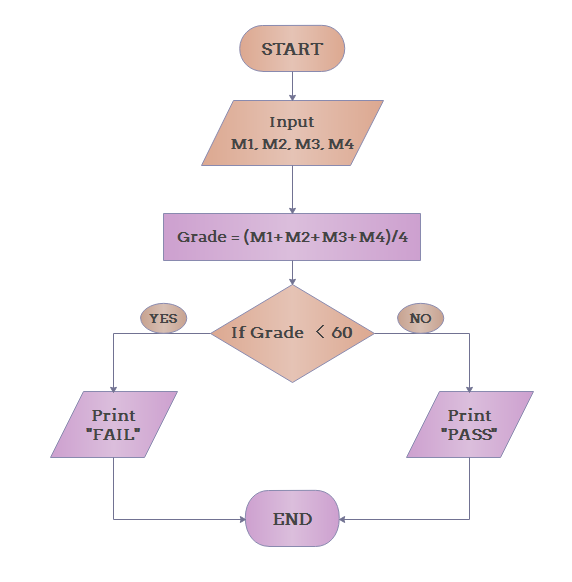Home
• Products
• Pricing
• Office Efficiency
• Project Management
• Graphic Design
• Engineering
• Education
• Special Industry
• Others

# What is Algorithm - Definition, Types and Application

An algorithm is a step-by-step demonstration of data processing or problem solving. This page will introduce the definition, types and applications of algorithm.

An algorithm can be described as a procedure or formula for problem solving. Algorithms can be widely used in various areas, computer programming, mathematics and daily lives. Then what is the definition of algorithm? How many types are there and in what ways can they be applied?

### Definition of Algorithm

Algorithm can be defined as "A sequence of steps to be carried out for a required output from a certain given input". There are 3 main features of algorithm from its definition:

1. The essential aim of an algorithm is to get a specific output,
2. An algorithm involves with several continuous steps,
3. The output comes after the algorithm finished the whole process.

So basically, all algorithms perform logically while following the steps to get an output for a given input.

### Types of Algorithm

Algorithms can be classified into 3 types based on their structures:

1. Sequence: this type of algorithm is characterized with a series of steps, and each step will be executed one after another.
2. Branching: this type of algorithm is represented by the "if-then" problems. If a condition is true, the output will be A, if the condition is false, the output will be B. This algorithm type is also known as "selection type".
3. Loop: for this type, the process might be repeatedly executed under a certain condition. It is represented by "while" and "for" problems. But make sure the process will end after a number of loops under the condition. This algorithm type is also known as "repetition type".

### Applications of Algorithm

As mentioned before, algorithms can be used in many areas, and they are often represented in flowchart form for visual understanding. In other words, a flowchart is a diagram that represents an algorithm, showing the steps in various boxes and displays the process by connecting the boxes together. Here are some examples for algorithm application in flowchart forms.

1. Algorithm Application for Math

Determine and Output Whether Number N is Even or Odd2. Algorithm Application for Computer Programming

Draw a flowchart for computing factorial N (N!)3. Algorithm Application for Daily Life

Determine Whether the Student Passed the Exam or NotThe examples above give a clear demonstration of the applicaitons of algorithms in math, computer programming and daily life. Creating a flowchart might be the best way to represent an algorithm. More examples please refer to the following links:

Explain Algorithm and Flowchart with Examples

Examples for Algorithm Flowcharts

Simple Algorithm Flowchart Template

## Get Started! You Will Love This Easy-To-Use Diagram Software.

Edraw Max is perfect not only for professional-looking flowcharts, organizational charts, mind maps, but also network diagrams, floor plans, workflows, fashion designs, UML diagrams, electrical diagrams, science illustration, charts and graphs... and that is just the beginning!